##### The Japanese Bonsai specialist
Direct order Contact Help / Services Newsletter# Hosta pocketful of sunshine

Hosta pocketful of sunshine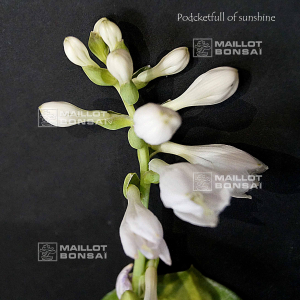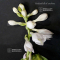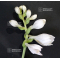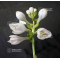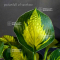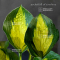ref. : 11083

12,00

Available quantity : 15Order

###### Description

Coming from a hybridization of Hosta Rainforest.

Beautiful variety coming from the United States.

Dimensions of the leaves :  about 13 x 11 cm.

This variety has round leaves, shiny clear green colored in the middle of the leaf and darker green colored on the sides.

White color flowers in summer.

Big rhizome delivered in a 1 liter plastic pot.

#about 3.5 #hosta 3.5 #pocketful 2.9 #sunshine 2.8 #variety 2.7 #colored 2.7 #hostas 2.6 #leaves 2.6 #coming 2.6 #green 2.5

###### Technical description
 height 30-50 cm

Formule
(( ROUND((CHAR_LENGTH(b.article_nom)-CHAR_LENGTH(REPLACE(b.article_nom, 'about', '')))/LENGTH('about')) + ROUND((CHAR_LENGTH(b.article_description)-CHAR_LENGTH(REPLACE(b.article_description, 'about', '')))/LENGTH('about')) ) * 3.5) + (( ROUND((CHAR_LENGTH(b.article_nom)-CHAR_LENGTH(REPLACE(b.article_nom, 'hosta', '')))/LENGTH('hosta')) + ROUND((CHAR_LENGTH(b.article_description)-CHAR_LENGTH(REPLACE(b.article_description, 'hosta', '')))/LENGTH('hosta')) ) * 3.5) + (( ROUND((CHAR_LENGTH(b.article_nom)-CHAR_LENGTH(REPLACE(b.article_nom, 'pocketful', '')))/LENGTH('pocketful')) + ROUND((CHAR_LENGTH(b.article_description)-CHAR_LENGTH(REPLACE(b.article_description, 'pocketful', '')))/LENGTH('pocketful')) ) * 2.9) + (( ROUND((CHAR_LENGTH(b.article_nom)-CHAR_LENGTH(REPLACE(b.article_nom, 'sunshine', '')))/LENGTH('sunshine')) + ROUND((CHAR_LENGTH(b.article_description)-CHAR_LENGTH(REPLACE(b.article_description, 'sunshine', '')))/LENGTH('sunshine')) ) * 2.8) + (( ROUND((CHAR_LENGTH(b.article_nom)-CHAR_LENGTH(REPLACE(b.article_nom, 'variety', '')))/LENGTH('variety')) + ROUND((CHAR_LENGTH(b.article_description)-CHAR_LENGTH(REPLACE(b.article_description, 'variety', '')))/LENGTH('variety')) ) * 2.7) + (( ROUND((CHAR_LENGTH(b.article_nom)-CHAR_LENGTH(REPLACE(b.article_nom, 'colored', '')))/LENGTH('colored')) + ROUND((CHAR_LENGTH(b.article_description)-CHAR_LENGTH(REPLACE(b.article_description, 'colored', '')))/LENGTH('colored')) ) * 2.7) + (( ROUND((CHAR_LENGTH(b.article_nom)-CHAR_LENGTH(REPLACE(b.article_nom, 'coming', '')))/LENGTH('coming')) + ROUND((CHAR_LENGTH(b.article_description)-CHAR_LENGTH(REPLACE(b.article_description, 'coming', '')))/LENGTH('coming')) ) * 2.6) + (( ROUND((CHAR_LENGTH(b.article_nom)-CHAR_LENGTH(REPLACE(b.article_nom, 'leaves', '')))/LENGTH('leaves')) + ROUND((CHAR_LENGTH(b.article_description)-CHAR_LENGTH(REPLACE(b.article_description, 'leaves', '')))/LENGTH('leaves')) ) * 2.6) + (( ROUND((CHAR_LENGTH(b.article_nom)-CHAR_LENGTH(REPLACE(b.article_nom, 'green', '')))/LENGTH('green')) + ROUND((CHAR_LENGTH(b.article_description)-CHAR_LENGTH(REPLACE(b.article_description, 'green', '')))/LENGTH('green')) ) * 2.5) + (( ROUND((CHAR_LENGTH(b.article_nom)-CHAR_LENGTH(REPLACE(b.article_nom, 'from', '')))/LENGTH('from')) + ROUND((CHAR_LENGTH(b.article_description)-CHAR_LENGTH(REPLACE(b.article_description, 'from', '')))/LENGTH('from')) ) * 2.4)

## Secure payment## Delivery

Our logistic partners :04 74 55 23 48
Pépinière MAILLOT-BONSAÏ
Le Bois Frazy
01990 RELEVANT - FRANCE
on appointmentThe Japanese Bonsai specialist
If you like Maillot Bonsaï, share on  facebook

Pensez à venir nous rendre visite sur rendez vous. 04 74 55 23 48 merci.

L'équipe Maillot bonsaï vous souhaite ses meilleurs vœux de bonheur, de santé et de réussite pour cette nouvelle année. C'est l'occasion pour nous de vous dire un IMMENSE MERCI d'avoir été à nos côtés cette année.

2023, c'est 365 jours où nous serons présents pour vous aider, vous guider et répondre à vos questions. Plus que jamais, nous continuerons à vous accompagner et à vous proposer des produits de qualité.   Cette nouvelle année sera riche en nouveautés, alors restez connecté pour ne rien rater !

Guy Maillot

OK